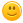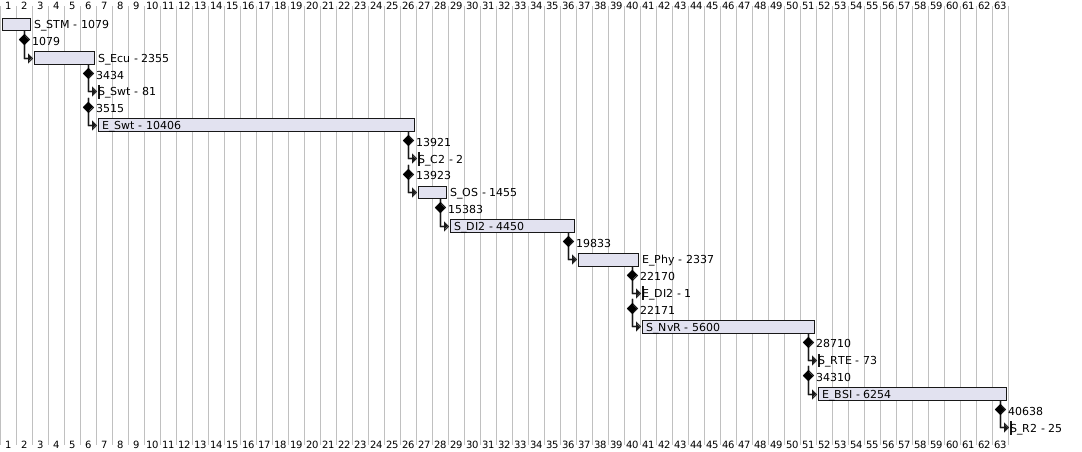# While Loop & Smarter Visualization of a System Startup

Dear PlantUML community,

I´m struggling with a nice visualization of a startup phase.

Input: json file which holds several snapshots. Each snapshot consist of a string identifier, a current timestamp and a delta timestamp

Output: a Gantt diagram which should show picture instead of pure numbers present in json file

My request to the experts:

1. Could you create a solution with a loop?
2. Could you bring into the current timestamp (=value from json file) into the diagram?
3. Could you make it possible that the loop is able to deal with different entries size of json file array?

Since I don´t found a way to attach files I add the json file and the diagram text here.

--- json file – start ----

```{
"log2" : [
{"Dvalue" : 83889,  "value" : 0, "str" : "S_STM"},
{"Dvalue" : 1079,   "value" : 1079, "str" : "S_Ecu"},
{"Dvalue" : 2355,   "value" : 3434, "str" : "S_Swt"},
{"Dvalue" : 81,     "value" : 3515, "str" : "E_Swt"},
{"Dvalue" : 10406,  "value" : 13921, "str" : "S_C2"},
{"Dvalue" : 2,      "value" : 13923, "str" : "S_OS"},
{"Dvalue" : 1455,   "value" : 15383, "str" : "S_DI2"},
{"Dvalue" : 4450,   "value" : 19833, "str" : "E_Phy"},
{"Dvalue" : 2337,   "value" : 22170, "str" : "E_DI2"},
{"Dvalue" : 1,      "value" : 22171, "str" : "S_NvR"},
{"Dvalue" : 5600,   "value" : 28710, "str" : "S_RTE"},
{"Dvalue" : 73,     "value" : 34310, "str" : "E_BSI"},
{"Dvalue" : 6254,   "value" : 40638, "str" : "S_R2"},
{"Dvalue" : 25,     "value" : 40664, "str" : "E_R2"}
]
}```

--- json file – end ----

--- diagram – start ----

```@startgantt
!function \$Scal(\$val)
!return (%intval(\$val) / 500)
!endfunction

!\$zero = \$STL.log2.str + " - " +  %string(\$STL.log2.Dvalue)
!\$one = \$STL.log2.str + " - " +  %string(\$STL.log2.Dvalue)
!\$two = \$STL.log2.str + " - " +  %string(\$STL.log2.Dvalue)
!\$three = \$STL.log2.str + " - " +  %string(\$STL.log2.Dvalue)
!\$four = \$STL.log2.str + " - " +  %string(\$STL.log2.Dvalue)
!\$five = \$STL.log2.str + " - " +  %string(\$STL.log2.Dvalue)
!\$six = \$STL.log2.str + " - " +  %string(\$STL.log2.Dvalue)
!\$seven = \$STL.log2.str + " - " +  %string(\$STL.log2.Dvalue)
!\$eight = \$STL.log2.str + " - " +  %string(\$STL.log2.Dvalue)
!\$nine = \$STL.log2.str + " - " +  %string(\$STL.log2.Dvalue)
!\$ten = \$STL.log2.str + " - " +  %string(\$STL.log2.Dvalue)
!\$eleven = \$STL.log2.str + " - " +  %string(\$STL.log2.Dvalue)
!\$twelve = \$STL.log2.str + " - " +  %string(\$STL.log2.Dvalue)

[\$zero] lasts \$Scal(\$STL.log2.Dvalue) days

[\$one] starts at [\$zero]'s end
[\$one] lasts \$Scal(\$STL.log2.Dvalue) days

[\$two] starts at [\$one]'s end
[\$two] lasts \$Scal(\$STL.log2.Dvalue) days

[\$three] starts at [\$two]'s end
[\$three] lasts \$Scal(\$STL.log2.Dvalue) days

[\$four] starts at [\$three]'s end
[\$four] lasts \$Scal(\$STL.log2.Dvalue) days

[\$five] starts at [\$four]'s end
[\$five] lasts \$Scal(\$STL.log2.Dvalue) days

[\$six] starts at [\$five]'s end
[\$six] lasts \$Scal(\$STL.log2.Dvalue) days

[\$seven] starts at [\$six]'s end
[\$seven] lasts \$Scal(\$STL.log2.Dvalue) days

[\$eight] starts at [\$seven]'s end
[\$eight] lasts \$Scal(\$STL.log2.Dvalue) days

[\$nine] starts at [\$eight]'s end
[\$nine] lasts \$Scal(\$STL.log2.Dvalue) days

[\$ten] starts at [\$nine]'s end
[\$ten] lasts \$Scal(\$STL.log2.Dvalue) days

[\$eleven] starts at [\$ten]'s end
[\$eleven] lasts \$Scal(\$STL.log2.Dvalue) days

[\$twelve] starts at [\$eleven]'s end
[\$twelve] lasts \$Scal(\$STL.log2.Dvalue) days
@endgantt```

--- diagram – end ----

answered Jun 8, 2022 by (55,740 points)

Hello A.,

Here is a proposal, using `%size` and `!while`/`!endwhile`:

```@startgantt

!function \$Scal(\$val)
!return (%intval(\$val) / 500)
!endfunction

!\$t=\$STL.log2.Dvalue
[\$STL.log2.str - \$t] as  lasts \$Scal(\$t) days

!\$size=%size(\$STL.log2)
!\$c=1
!while \$c <= \$size-2
!\$b=\$c-1
!\$d=\$c+1
!\$t=\$STL.log2[\$d].Dvalue
[\$STL.log2[\$c].str - \$t] as [\$c] starts at [\$b]'s end and lasts \$Scal(\$t) days
!\$c=\$c+1
!endwhile

@endgantt```

Here is the result:Regards.

commented Jun 9, 2022 by (160 points)

HI hi The-Lu,

what else could I say than thanks very much. Sadly I´m still not that deep in PlantUML syntax but I was able to extend your gantt proposal with the current time snapshot.

Amazing the support within this community@startgantt
!\$STL = {
"log2" : [
{"Dvalue" : 83889,  "value" : 0, "str" : "S_STM"},
{"Dvalue" : 1079,   "value" : 1079, "str" : "S_Ecu"},
{"Dvalue" : 2355,   "value" : 3434, "str" : "S_Swt"},
{"Dvalue" : 81,     "value" : 3515, "str" : "E_Swt"},
{"Dvalue" : 10406,  "value" : 13921, "str" : "S_C2"},
{"Dvalue" : 2,      "value" : 13923, "str" : "S_OS"},
{"Dvalue" : 1455,   "value" : 15383, "str" : "S_DI2"},
{"Dvalue" : 4450,   "value" : 19833, "str" : "E_Phy"},
{"Dvalue" : 2337,   "value" : 22170, "str" : "E_DI2"},
{"Dvalue" : 1,      "value" : 22171, "str" : "S_NvR"},
{"Dvalue" : 5600,   "value" : 28710, "str" : "S_RTE"},
{"Dvalue" : 73,     "value" : 34310, "str" : "E_BSI"},
{"Dvalue" : 6254,   "value" : 40638, "str" : "S_R2"},
{"Dvalue" : 25,     "value" : 40664, "str" : "E_R2"}
]
}

!function \$Scal(\$val)
!return (%intval(\$val) / 500)
!endfunction

!\$t=\$STL.log2.Dvalue
[\$STL.log2.str - \$t] as  lasts \$Scal(\$t) days

!\$size=%size(\$STL.log2)
!\$c=1
!while \$c <= \$size-2
!\$b=\$c-1
!\$d=\$c+1
!\$t=\$STL.log2[\$d].Dvalue
[\$STL.log2[\$c].value] happens at [\$b]'s end
[\$STL.log2[\$c].str - \$t] as [\$c] starts at [\$b]'s end and lasts \$Scal(\$t) days
!\$c=\$c+1
!endwhile

@endgantt

--------------------------------------------------------